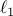# Compressed Sensing with Quantized Measurements

A. Zymnis, S. Boyd, and E. Candes

IEEE Signal Processing Letters, 17(2):149-152, February 2010.

We consider the problem of estimating a sparse signal from a set of quantized, Gaussian noise corrupted measurements, where each measurement corresponds to an interval of values. We give two methods for (approximately) solving this problem, each based on minimizing a differentiable convex function plus anregularization term. Using a first order method developed by Hale et al, we demonstrate the performance of the methods through numerical simulation. We find that, using these methods, compressed sensing can be carried out even when the quantization is very coarse, e.g., 1 or 2 bits per measurement.